Courses

# Metallurgical Engineering - MT 2016 GATE Paper (Practice Test)

## 65 Questions MCQ Test GATE Past Year Papers for Practice (All Branches) | Metallurgical Engineering - MT 2016 GATE Paper (Practice Test)

Description
This mock test of Metallurgical Engineering - MT 2016 GATE Paper (Practice Test) for GATE helps you for every GATE entrance exam. This contains 65 Multiple Choice Questions for GATE Metallurgical Engineering - MT 2016 GATE Paper (Practice Test) (mcq) to study with solutions a complete question bank. The solved questions answers in this Metallurgical Engineering - MT 2016 GATE Paper (Practice Test) quiz give you a good mix of easy questions and tough questions. GATE students definitely take this Metallurgical Engineering - MT 2016 GATE Paper (Practice Test) exercise for a better result in the exam. You can find other Metallurgical Engineering - MT 2016 GATE Paper (Practice Test) extra questions, long questions & short questions for GATE on EduRev as well by searching above.
QUESTION: 1

Solution:
QUESTION: 2

Solution:
QUESTION: 3

### Choose the most appropriate set of words from the options given below to complete the following sentence. _________ ___________ is a will, _________ is a way.

Solution:
QUESTION: 4

(x % of y) + (y % of x) is equivalent to _______________.

Solution:
QUESTION: 5

The sum of the digits of a two digit number is 12. If the new number formed by reversing the digits is greater than the original number by 54, find the original number.

Solution:
QUESTION: 6

Q. 6 – Q. 10 carry two marks each.

Two finance companies, P and Q, declared fixed annual rates of interest on the amounts invested with them. The rates of interest offered by these companies may differ from year to year. Year-wise annual rates of interest offered by these companies are shown by the line graph provided below.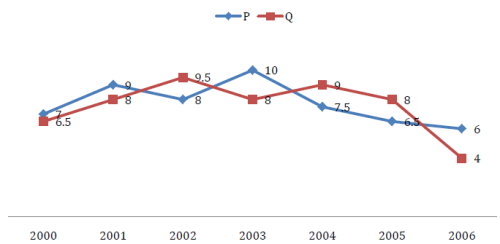If the amounts invested in the companies, P and Q, in 2006 are in the ratio 8:9, then the amounts received after one year as interests from companies P and Q would be in the ratio:

Solution:
QUESTION: 7

Today, we consider Ashoka as a great ruler because of the copious evidence he left behind in the form of stone carved edicts. Historians tend to correlate greatness of a king at his time with the availability of evidence today.

Which of the following can be logically inferred from the above sentences?

Solution:
QUESTION: 8

Fact 1: Humans are mammals. Fact 2: Some humans are engineers. Fact 3: Engineers build houses. If the above statements are facts, which of the following can be logically inferred?

I. All mammals build houses.
II. Engineers are mammals.
III. Some humans are not engineers.

Solution:
QUESTION: 9

A square pyramid has a base perimeter x, and the slant height is half of the perimeter. What is the lateral surface area of the pyramid?

Solution:
QUESTION: 10

Ananth takes 6 hours and Bharath takes 4 hours to read a book. Both started reading copies of the book at the same time. After how many hours is the number of pages to be read by Ananth, twice that to be read by Bharath? Assume Ananth and Bharath read all the pages with constant pace.

Solution:
*Answer can only contain numeric values
QUESTION: 11

Useful Data:
Gas constant (R) 8.314 J.mol-1K-1
Acceleration due to gravity (g) 9.81 m2.s-1
Atomic weight of oxygen 16 g.mol-1
Atomic weight of silicon 28 g.mol-1
Atomic weight of iron 56 g.mol-1

Q. 11 – Q. 35 carry one mark each.

For the transformation shown below, if one of the eigenvalues is 6, the other eigenvalue of the matrix is ______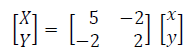Solution:
QUESTION: 12

The solution of the differential equation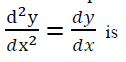[where, C, C1 and C2 are constants]

Solution:
QUESTION: 13

If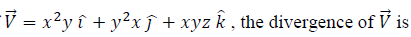Solution:
QUESTION: 14

The first law of thermodynamics can be stated as

[where, E, Q and W denote internal energy, heat and work, respectively]

Solution:
QUESTION: 15

In a typical Ellingham diagram for the oxides, the C + O2 = CO2 line is nearly horizontal because

Solution:
QUESTION: 16

Activation energy of a chemical reaction, homogeneous or heterogeneous, is graphically estimated from a plot between

[where, k is the rate constant and T is the absolute temperature]

Solution:
QUESTION: 17

The passive film in stainless steel forms above the

Solution:
QUESTION: 18

During the roasting of a sulfide ore of a metal M, the possible solid phases are M, MS, MO and MSO4. Assuming that both SO2 and O2 are always present in the roaster, the solid phases that can co-exist at thermodynamic equilibrium are

Solution:
QUESTION: 19

Match the entities in Column I with the corresponding processes in Column II.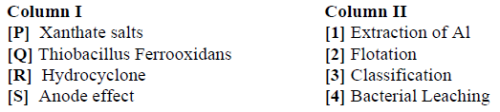Solution:

Explanation : a) Sodium ethyl xanthate is used in the mining industry as a flotation agent. A closely related potassium ethyl xanthate (KEX) is obtained as the anhydrous salt.

b) Thiobacillus ferrooxidans is a gram-negative, highly acidophilic (pH 1.5 to 2.0), autotrophic bacterium that obtains its energy through the oxidation of ferrous iron or reduced inorganic sulfur compounds.

c) A hydrocyclone is a classifier that has two exits on the axis: one on the bottom (underflow or reject) and one at the top (overflow or accept). The underflow is generally the denser or coarser fraction, while the overflow is the lighter or finer fraction.

d) During an anode effect the normal reactions that produce aluminum are interrupted and other electrochemical reactions take place with the formations of gases CO, CF4 and C2F6. These gases forms bubbles that adhere to the anode bottom, creating an electrical insulating layer which is the reason why the voltage of the cell increases up to 10 – 50 volts and even more. The maximum voltage reached during the anode effects depends also by the bath level. With high bath levels the maximum voltage reached is lower because some fraction of the line current passes through the sides of the anodes, free from the adhering bubbles.

QUESTION: 20

A sub-lance is used to monitor composition and temperature in

Solution:
QUESTION: 21

The chemical formula of wüstite is

Solution:
*Answer can only contain numeric values
QUESTION: 22

The lattice parameter of face-centered cubic iron (γ-Fe) is 0.3571 nm. The radius (in nm) of the octahedral void in γ-Fe is ___________

Solution:
QUESTION: 23

For an ideal hexagonal-closed packed structure, the c/a ratio and packing efficiency respectively are

Solution:
QUESTION: 24

A schematic of X-ray diffraction pattern of a single phase cubic polycrystal is given below. The miller indices of peak A is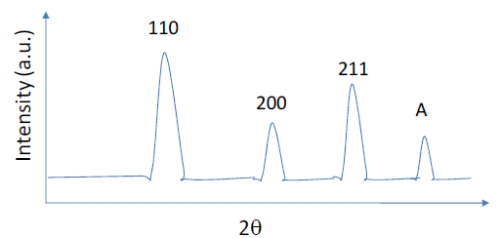Solution:
QUESTION: 25

Which of the following cooling curves (shown in schematic) in an eutectoid steel will produce 50% bainitic structure?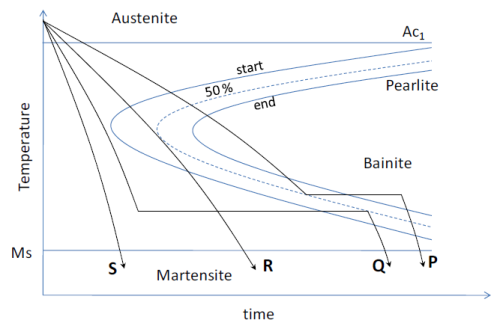Solution:
*Answer can only contain numeric values
QUESTION: 26

The Burger’s vector of a dislocation in a cubic crystal (with lattice parameter a) is a/2(110) and dislocation line is along  direction. The angle (in degrees) between the dislocation line and its Burger’s vector is_______

Solution:
*Answer can only contain numeric values
QUESTION: 27

For the tensile stress-strain curve of a material shown in the schematic, the resilience (in MPa) is _________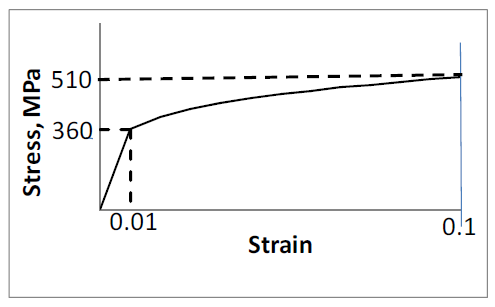Solution:
QUESTION: 28

A plastically deformed metal crystal at low temperature exhibits wavy slip line pattern due to

Solution:
QUESTION: 29

Creep resistance decreases due to

Solution:
QUESTION: 30

The operation NOT associated with casting is

Solution:
QUESTION: 31

Of the following welding processes

[P] Laser Beam Welding

[Q] Submerged Arc Welding

[R] Metal Inert Gas Welding

the width of the heat-affected zone in decreasing order is

Solution:
QUESTION: 32

For dye-penetrant test, identify the CORRECT statement

Solution:
*Answer can only contain numeric values
QUESTION: 33

Aluminium powder having an apparent density of 810 kg.m-3 is compacted in a cylindrical die at 600 MPa. The density of the as-pressed aluminium compact is 1755 kg.m-3. If the height of the as-pressed compact is 12 mm, the fill height (in mm) required is ___________

Solution:
*Answer can only contain numeric values
QUESTION: 34

A rolling mill has a roll diameter of 200 mm. If coefficient of friction is 0.1, then the maximum possible reduction (in mm) during rolling of a 250 mm thick plate is ___________

Solution:
*Answer can only contain numeric values
QUESTION: 35

A hot body cools according to the following equation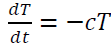where, T is the instantaneous temperature at time t, and the constant c = 0.05 s-1. Reduce the differential equation into its finite difference form using forward difference. For maintaining numerical stability, the maximum value of the time step Δt (in seconds) is ________

Solution:
*Answer can only contain numeric values
QUESTION: 36

Q. 36 – Q. 65 carry two marks each.

Solve the equation x= e-x using Newton-Raphson method. Starting with an initial guess value x0 = 0, the value of x after the first iteration is ____________

Solution:
*Answer can only contain numeric values
QUESTION: 37

A coin is tossed three times. It is known that out of the three tosses, one is a HEAD. The probability of the other two tosses also being HEADs is _____________

Solution:
QUESTION: 38

The vector parallel to the plane 3x - 2y + z = -1 is

Solution:
*Answer can only contain numeric values
QUESTION: 39

The value of the integral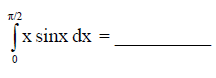Solution:
QUESTION: 40

The grain sizes (in μm) measured at five locations in an alloy sample are: 16, 14, 18, 15 and 13. The mean, median and standard deviation of grain sizes respectively are (in μm)

Solution:
*Answer can only contain numeric values
QUESTION: 41

The change of standard state from pure liquid to 1 wt.% for Si dissolved in liquid Fe at 1873 K is expressed as

Si (liq.) = Si (1 wt.%)

Given that the activity coefficient of Si at infinite dilution in Fe is 10-3, the standard Gibbs free energy change (in kJ) for this equilibrium is_____________

Solution:
*Answer can only contain numeric values
QUESTION: 42

The following experimental data are available for a hypothetical binary liquid system A-B at 1073 K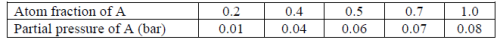When the atom fraction of A is 0.4, the activity of A in the liquid is ______________

Solution:
*Answer can only contain numeric values
QUESTION: 43

The lining of a box-type furnace is made up of a refractory layer and steel plate as shown in the figure. Steady state temperature at the surface of the refractory is 1273 K and that at the outer steel surface is 473 K. If the steady-state heat flux through the refractory-steel plate composite is 1600 W.m-2, and heat flow is along x-direction, the thermal contact resistance (W-1.m2.K) between refractory and steel is _____________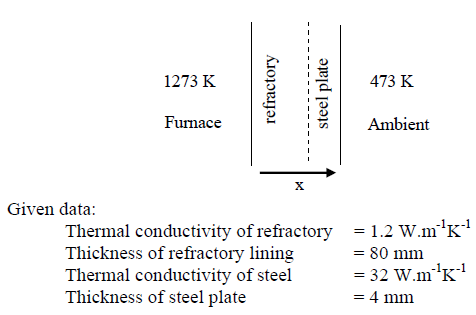Solution:
*Answer can only contain numeric values
QUESTION: 44

The height of a liquid metal column in a cylindrical vessel is 3.2 m. At time t=0, liquid metal is drained out from the vessel through a small nozzle located at the base of the vessel. Neglecting frictional losses, the initial mass flow rate (in kg.s-1) through the nozzle is ________________

Given data:
Density of liquid metal = 7000 kg.m-3
Nozzle diameter = 30 mm
Nozzle discharge coefficient = 0.80

Solution:
QUESTION: 45

Match entities listed in Column I with their correct dimensions given in Column II: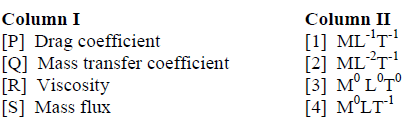Solution:
*Answer can only contain numeric values
QUESTION: 46

Direct Reduced Iron (DRI) produced from a gas based process contains Fe, FeO, C and remainder being gangue. The chemical composition of DRI is: Total Fe = 92 wt.% and Metallic Fe = 84 wt.%. The weight percent of FeO in DRI is _________________

Solution:
*Answer can only contain numeric values
QUESTION: 47

Mould heat flux (qm) for billet casters is expressed (in SI unit) as a function of distance below the meniscus (z)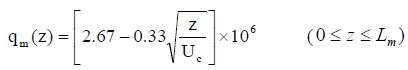If mould length (Lm) is 0.8 m and casting speed (Uc) is 0.2 m.s-1, the average mould flux (in MW.m-2) is ________________

Solution:
*Answer can only contain numeric values
QUESTION: 48

In BOF steelmaking, 5 metric ton of lime containing 90 wt.% CaO is used to refine 100 metric ton of hot metal containing 93.2 wt.% Fe. The slag produced during refining contains 40 wt.% CaO and 22 wt.% FeO. Neglecting material losses, the yield of Fe (in %) is _________________

Solution:
*Answer can only contain numeric values
QUESTION: 49

In vacuum degassing of steel, 14 ppm of dissolved nitrogen is in equilibrium with 1 mbar of nitrogen gas at 1873 K. At the same temperature, if the pressure is lowered to 0.7 mbar, the equilibrium nitrogen content (in ppm) is _____________

Solution:
*Answer can only contain numeric values
QUESTION: 50

During isothermal phase transformation (in solid-state), fraction transformed is measured at two different transformation times: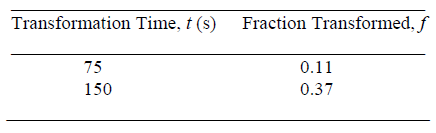Assuming Avrami kinetics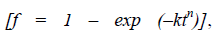the fraction transformed in 300 seconds is _______________

Solution:
QUESTION: 51

Zinc oxide is reduced at a constant temperature in a closed reactor using ZnO(s) and C(s) as the only starting materials. The following reactions are assumed to be at thermodynamic equilibrium:

ZnO(s) + C(s) = Zn(g) + CO(g)

2CO(g) = CO2(g) + C(s)

Assume ideal gas behaviour. Based on mole balance, the relationship applicable to the system at equilibrium is

Solution:
QUESTION: 52

The critical nucleus size (in nm) when copper melt is under-cooled by 100 K isSolution:
QUESTION: 53

The density and corresponding crystallinity of two poly-propylene material are given below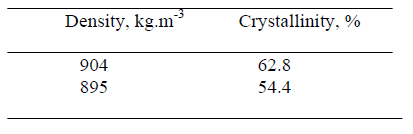The density of totally amorphous poly-propylene (in kg.m-3) is:

Solution:
QUESTION: 54

A simplified energy band-diagram of an intrinsic semiconductor at thermal equilibrium (300 K) is shown. In the accompanying table, which one of the four columns correctly represents the listed parameters? Assume same effective mass for electrons and holes.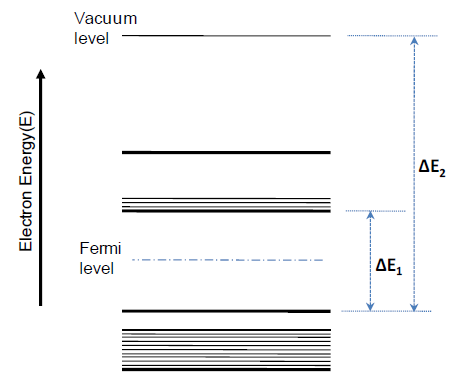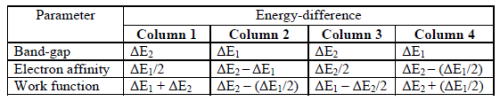Solution:
*Answer can only contain numeric values
QUESTION: 55

A binary phase diagram is shown in the schematic.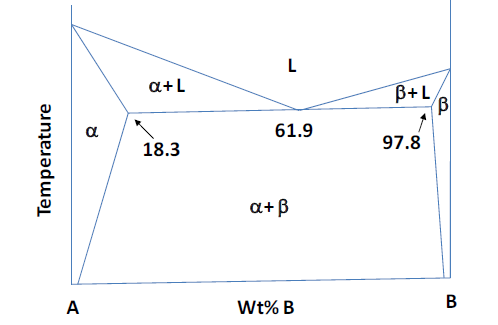Upon complete solidification of a binary alloy system A-B, the fraction of pro-eutectic α- phase present is 0.50. The alloy composition in terms of wt%. B is _________________

Solution:
*Answer can only contain numeric values
QUESTION: 56

Fatigue behaviour of an aluminium alloy is shown in the S-N plot. A piston rod made of this material is subjected to: (i) 1000 cycles at 420 MPa, followed by (ii) 1000 cycles at 300 MPa. Using Miner’s rule of cumulative damage, the remaining fatigue life (in terms of number of cycles) at stress of 250 MPa is _______________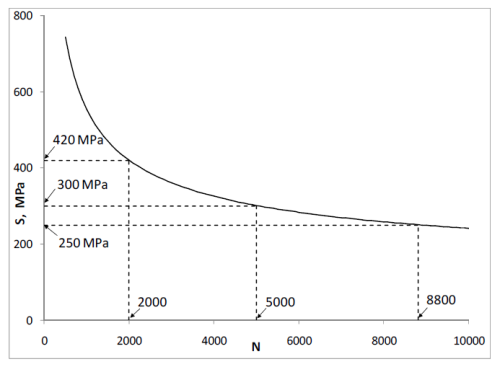Solution:
*Answer can only contain numeric values
QUESTION: 57

A glass plate has two parallel cracks. One of them is an internal crack of length 5 μm and the other is a surface crack of length 3 μm. A tensile stress is applied perpendicular to the crack surfaces. The fracture stress (in MPa) is ___________

Given data (for glass plate):
Young’s Modulus = 70 GPa
Surface energy per unit area = 1 J.m-2

Solution:
*Answer can only contain numeric values
QUESTION: 58

A tensile stress is applied along the  direction in a FCC metal crystal. The critical resolved shear stress is 6 MPa. The tensile stress (in MPa) required for initiating slip on the (111) slip plane is_____________

Solution:
*Answer can only contain numeric values
QUESTION: 59

For a bcc metal the ratio of the surface energy per unit area of the (100) plane to that of the (110) plane is ______________

Solution:
QUESTION: 60

For a polymer reinforced with 40 vol.% glass fiber, the elastic modulus (in GPa) along the transverse direction is

[Eglass fiber = 70 GPa; Epolymer = 3.5 GPa]

Solution:
*Answer can only contain numeric values
QUESTION: 61

In a sand-mould, a sprue of 0.25 m height and a top cross-section area of 2.2 m2 is provided to maintain the melt flow rate at 4 m3.s-1. To prevent aspiration of molten metal, the maximum cross-section area (in m2) at the base of the sprue is ____________

Solution:
QUESTION: 62

For casting a cylindrical aluminum bloom having a length of 1000 mm and diameter of 750 mm, the approximate solidification time (in minutes) estimated using Chvorinov’s rule is

[The mould constant is 2 s/mm2]

Solution:
QUESTION: 63

A liquid phase sintered SiC-Ni composite has a solid-solid grain boundary energy (γSiC-SiC) of 0.80 J.m-2 and a solid-liquid (γSiC-Ni) interfacial energy of 0.45 J.m-2. For a SiC grain size of 20 μm, the average interparticle (SiC-SiC) neck size (in μm) is:

Solution:
QUESTION: 64

Match the deformation processes in Column I with the corresponding stress states listed in Column II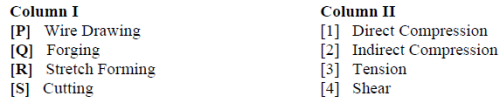Solution:
QUESTION: 65

Railway tracks are typically manufactured using

Solution: